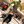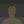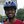0%
0%

# Scientific Calculator using evive

fully functional scientific calculator using evive

Similar projects worth following
943 views
DIY projects are amazing and if they are of regular use then they are awesome to make.
We have built a Scientific calculator having lots of functions like

Basic algebraic operations (+, -, *, %)
Trigonometric functions (SINe, COSine, TANgent)
Inverse trigonometric functions (arcSin, arcCos, arcTan)
Logarithmic function
Square root.

An arduino sketch is made to implement this. It calculates accurately upto six decimal places and display upto four decimal place although you can increase it upto 6 also.

• 1
Step 1

Firstly we will make a simple calculator that does simple operations like addition, subtraction, multiplication and division. We have used the A, B, C, D keys for the above operations respectively. For the 'equal to' operation we have used '#' key and for 'clear the screen' we have used '*' key in the keypad. In the attached image, connections for keypad are mentioned.

For the coding part we have taken help from the already build code at the arduino site-

Rest is just tweaking it to run by changing the pins etc. We have modified the code for 1.8" TFT (ST7735R).

But this was very simple!! Lets go ahead

• 2
Step 2

For both our input numbers the algorithm is basically the same.

Let the first number be a double named 'first'. We initialize it to be zero.

We make a Boolean 'isDecimal' and initialize it as false. This means that unless the decimal point is given as an input, the number is not a decimal.

Last, declare a float 'decimals' and set it as 10.0. We will use it for keeping a counter of our place after the decimal point.

Now if isDecimal is false, it means the number is not yet a decimal. Suppose you are storing your input number from the keypad as key.

You just need to update first=first*10+key.

But if isDecimal is true, the number is a decimal. You need to now update as

first=first+key/decimals, and decimals=decimals*10.

We keep repeating the above steps until the input for some operation is detected. Then we similarly detect the second number. Using the knowledge of the operation called, we operate the numbers and print the result when '=' is detected.

Remember to restate the values of decimals=10.0 and isDecimal=false after detection for a number is complete.

``` if(digitalRead(17)==HIGH)
{
delay(250);
{ tone(buzzer,5000,100);
isDecimal1=true;
}
}
if(customKey!=NO_KEY){
tone(buzzer,5000,100);
Serial.println(isDecimal1);
switch(customKey)
{
case '0' ... '9': // This keeps collecting the first value until a operator is pressed "+-"
if (isDecimal1==false)
{
lcd.setCursor(0,0);
first = first * 10 + (customKey - '0');
lcd.print(first,4);
}
else
{
lcd.setCursor(0,0);
first=first+(customKey - '0')/decimals1;
decimals1=decimals1*10;
lcd.print(first,4);
}```

• 3
Step 3

Now, we have to add more buttons for the scientific functionality. We have used some colorful buttons as shown in figure. Now assign them accordingly. Take care to assign similar buttons to similar functions.

Since evive uses Arduino Mega, we have used following pins:

• sine=Pin25
• cosine=Pin27
• tangent=Pin23
• log=Pin22
• arcsin=Pin24
• arccos=Pin26
• arctan=Pin14
• root=Pin15

Share

## Similar Projects

Project Owner Contributor

### Arduino based tank project for kidsHulk

Project Owner Contributor

### Automatic targeting system using scrap arduino+IAghzatomic

Project Owner Contributor

### evive: a prototyping platform for makersDhrupal R Shah

# Does this project spark your interest?

Become a member to follow this project and never miss any updates